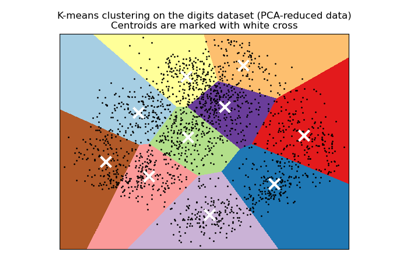# sklearn.preprocessing.scale¶

sklearn.preprocessing.scale(X, axis=0, with_mean=True, with_std=True, copy=True)[source]

Standardize a dataset along any axis

Center to the mean and component wise scale to unit variance.

Read more in the User Guide.

Parameters: X : {array-like, sparse matrix} The data to center and scale. axis : int (0 by default) axis used to compute the means and standard deviations along. If 0, independently standardize each feature, otherwise (if 1) standardize each sample. with_mean : boolean, True by default If True, center the data before scaling. with_std : boolean, True by default If True, scale the data to unit variance (or equivalently, unit standard deviation). copy : boolean, optional, default True set to False to perform inplace row normalization and avoid a copy (if the input is already a numpy array or a scipy.sparse CSC matrix and if axis is 1).

StandardScaler
Performs scaling to unit variance using theTransformer API (e.g. as part of a preprocessing sklearn.pipeline.Pipeline).

Notes

This implementation will refuse to center scipy.sparse matrices since it would make them non-sparse and would potentially crash the program with memory exhaustion problems.

Instead the caller is expected to either set explicitly with_mean=False (in that case, only variance scaling will be performed on the features of the CSC matrix) or to call X.toarray() if he/she expects the materialized dense array to fit in memory.

To avoid memory copy the caller should pass a CSC matrix.

NaNs are treated as missing values: disregarded to compute the statistics, and maintained during the data transformation.

We use a biased estimator for the standard deviation, equivalent to numpy.std(x, ddof=0). Note that the choice of ddof is unlikely to affect model performance.

For a comparison of the different scalers, transformers, and normalizers, see examples/preprocessing/plot_all_scaling.py.

## Examples using sklearn.preprocessing.scale¶A demo of K-Means clustering on the handwritten digits data